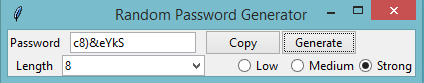# Python | Random Password Generator using Tkinter

With growing technology, everything has relied on data and securing these data is the main concern. Passwords are meant to keep the data safe that we upload on the Internet.
An easy password can be hacked easily and all the personal information can be misused. In order to prevent such things and keep the data safe, it is quite necessary to keep our passwords very strong.

Let’s create a simple application which can randomly generate strong passwords using Python `Tkinter `module.

This application can generate random password, with the combination of letters, numerics, and special characters. One can mention length of the password based on requirement and can also select the strength of the password.

Modules needed:

```import random
import pyperclip
from tkinter import * from tkinter.ttk import *
```

Below is the implementation:

 `# Python program to generate random ` `# password using Tkinter module ` `import` `random ` `import` `pyperclip ` `from` `tkinter ``import` `*` `from` `tkinter.ttk ``import` `*` ` `  `# Function for calculation of password ` `def` `low(): ` `    ``entry.delete(``0``, END) ` ` `  `    ``# Get the length of passowrd ` `    ``length ``=` `var1.get() ` ` `  `    ``lower ``=` `"abcdefghijklmnopqrstuvwxyz"` `    ``upper ``=` `"ABCDEFGHIJKLMNOPQRSTUVWXYZabcdefghijklmnopqrstuvwxyz"` `    ``digits ``=` `"ABCDEFGHIJKLMNOPQRSTUVWXYZabcdefghijklmnopqrstuvwxyz0123456789 !@#\$%^&*()"` `    ``password ``=` `"" ` ` `  `    ``# if strength selected is low ` `    ``if` `var.get() ``=``=` `1``: ` `        ``for` `i ``in` `range``(``0``, length): ` `            ``password ``=` `password ``+` `random.choice(lower) ` `        ``return` `password ` ` `  `    ``# if strength selected is medium ` `    ``elif` `var.get() ``=``=` `0``: ` `        ``for` `i ``in` `range``(``0``, length): ` `            ``password ``=` `password ``+` `random.choice(upper) ` `        ``return` `password ` ` `  `    ``# if strength selected is strong ` `    ``elif` `var.get() ``=``=` `3``: ` `        ``for` `i ``in` `range``(``0``, length): ` `            ``password ``=` `password ``+` `random.choice(digits) ` `        ``return` `password ` `    ``else``: ` `        ``print``(``"Please choose an option"``) ` ` `  ` `  `# Function for generation of password ` `def` `generate(): ` `    ``password1 ``=` `low() ` `    ``entry.insert(``10``, password1) ` ` `  ` `  `# Function for copying password to clipboard ` `def` `copy1(): ` `    ``random_password ``=` `entry.get() ` `    ``pyperclip.copy(random_password) ` ` `  ` `  `# Main Function ` ` `  `# create GUI window ` `root ``=` `Tk() ` `var ``=` `IntVar() ` `var1 ``=` `IntVar() ` ` `  `# Title of your GUI window ` `root.title(``"Random Password Generator"``) ` ` `  `# create label and entry to show ` `# password generated ` `Random_password ``=` `Label(root, text``=``"Password"``) ` `Random_password.grid(row``=``0``) ` `entry ``=` `Entry(root) ` `entry.grid(row``=``0``, column``=``1``) ` ` `  `# create label for length of password ` `c_label ``=` `Label(root, text``=``"Length"``) ` `c_label.grid(row``=``1``) ` ` `  `# create Buttons Copy which will copy ` `# password to clipboard and Generate ` `# which will generate the password ` `copy_button ``=` `Button(root, text``=``"Copy"``, command``=``copy1) ` `copy_button.grid(row``=``0``, column``=``2``) ` `generate_button ``=` `Button(root, text``=``"Generate"``, command``=``generate) ` `generate_button.grid(row``=``0``, column``=``3``) ` ` `  `# Radio Buttons for deciding the ` `# strength of password ` `# Default strength is Medium ` `radio_low ``=` `Radiobutton(root, text``=``"Low"``, variable``=``var, value``=``1``) ` `radio_low.grid(row``=``1``, column``=``2``, sticky``=``'E'``) ` `radio_middle ``=` `Radiobutton(root, text``=``"Medium"``, variable``=``var, value``=``0``) ` `radio_middle.grid(row``=``1``, column``=``3``, sticky``=``'E'``) ` `radio_strong ``=` `Radiobutton(root, text``=``"Strong"``, variable``=``var, value``=``3``) ` `radio_strong.grid(row``=``1``, column``=``4``, sticky``=``'E'``) ` `combo ``=` `Combobox(root, textvariable``=``var1) ` ` `  `# Combo Box for length of your password ` `combo[``'values'``] ``=` `(``8``, ``9``, ``10``, ``11``, ``12``, ``13``, ``14``, ``15``, ``16``, ` `                   ``17``, ``18``, ``19``, ``20``, ``21``, ``22``, ``23``, ``24``, ``25``, ` `                   ``26``, ``27``, ``28``, ``29``, ``30``, ``31``, ``32``, ``"Length"``) ` `combo.current(``0``) ` `combo.bind(``'<>'``) ` `combo.grid(column``=``1``, row``=``1``) ` ` `  `# start the GUI ` `root.mainloop() `

Output:My Personal Notes arrow_drop_upCheck out this Author's contributed articles.

If you like GeeksforGeeks and would like to contribute, you can also write an article using contribute.geeksforgeeks.org or mail your article to contribute@geeksforgeeks.org. See your article appearing on the GeeksforGeeks main page and help other Geeks.

Please Improve this article if you find anything incorrect by clicking on the "Improve Article" button below.

Article Tags :

3

Please write to us at contribute@geeksforgeeks.org to report any issue with the above content.